• 实现组合绘图、个图形重合叠加、图片叠加绘图。不同的数据放到同一张图展示。
不同的数据放到同一张图展示
par(new=TRUE)  # 每叠加一次新图形，运行一次该程序命令，即可实现在原图上继续叠加数据绘图
https://blog.csdn.net/tandelin/article/details/94362055
plot(x1,x2,xlab="维度",ylab="物种多样性数量");    #做散点图
lines(lowess(x1,x2),col=2);    #利用lowess做回归曲线
par(new=TRUE)  # 是否叠加新图形，没叠加一次运行一次该命令
plot(x3,x4,yaxt="n",xaxt="n",xlab="维度",ylab="物种多样性数量");    #做散点图
lines(lowess(x1,x2),col=2,lty=4);    #利用lowess做回归曲线

图形叠加后效果如下：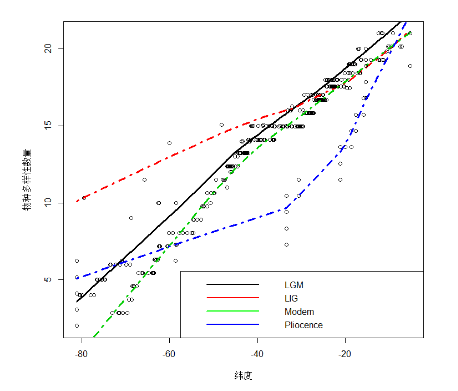不同的图叠加拼凑在一起展示
用grid.newpage()函数命令选择是否进行图形叠加，该命令有先后顺序，先运行。
library(grid)
library(ggplot2)
gg <- ggplot(mpg, aes(displ, hwy, colour = class)) +  geom_point()
library(png)
logo <- readPNG("C:\\Users\\TD\\Desktop\\test.png")
grid.newpage()
print(gg)  # 绘制第一个图
vp <- viewport(x = 0.6, y = 0.3, width = 0.2, height = 0.2)
grid.raster(logo, vp = vp)  # 将第一个图和第二个图形合并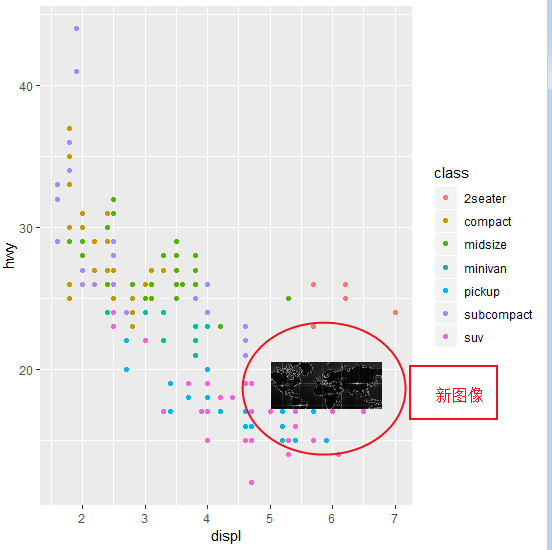展开全文• par(mfrow=c(2,2))，可以理解将绘图区域分割为2x2的矩阵区域，另可参照《R语言实战》3.5图形的组合 ##################par(mfrow=c(2,2))#################### attach(iris) opar <- par(no.readonly = TRUE) ...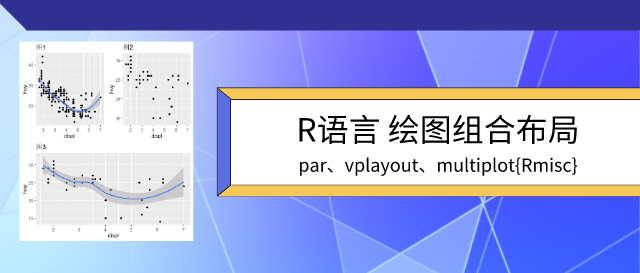​

01

—

图形组合布局

par(mfrow=c(2,2))

par(mfrow=c(2,2))，可以理解将绘图区域分割为2x2的矩阵区域，另可参照《R语言实战》3.5图形的组合

##################par(mfrow=c(2,2))####################

attach(iris)

opar <- par(no.readonly = TRUE)

# data(iris)

# head(iris)

# > head(iris)

# Sepal.Length Sepal.Width Petal.Length Petal.Width Species

# 1          5.1         3.5          1.4         0.2  setosa

# 2          4.9         3.0          1.4         0.2  setosa

# 3          4.7         3.2          1.3         0.2  setosa

# 4          4.6         3.1          1.5         0.2  setosa

# 5          5.0         3.6          1.4         0.2  setosa

# 6          5.4         3.9          1.7         0.4  setosa

​par(mfrow=c(2,2)) # 设置2x2的布局

plot(Sepal.Length,Sepal.Width, main = "第一张图")

boxplot(Sepal.Length, horizontal = TRUE, main = "第二张图")

boxplot(Sepal.Width, main = "第三张图")

hist(Petal.Length, main = "第四张图")​

par(opar)

detach(iris)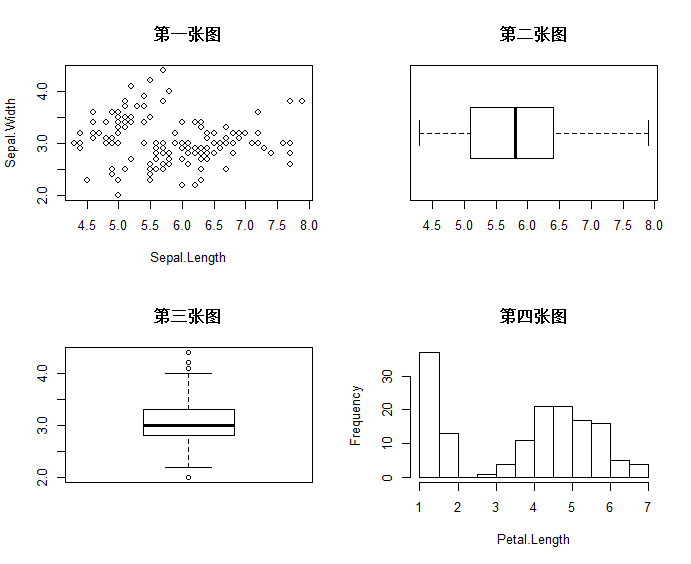02

—

图形组合布局

par(fig=c(x1, x2, y1, y2), new = TRUE)

par(fig=c(x1, x2, y1, y2), new = TRUE)，取x1,x2,y1,y2四条线圈住的位置绘图图形，另可参照《R语言实战》3.5图形的组合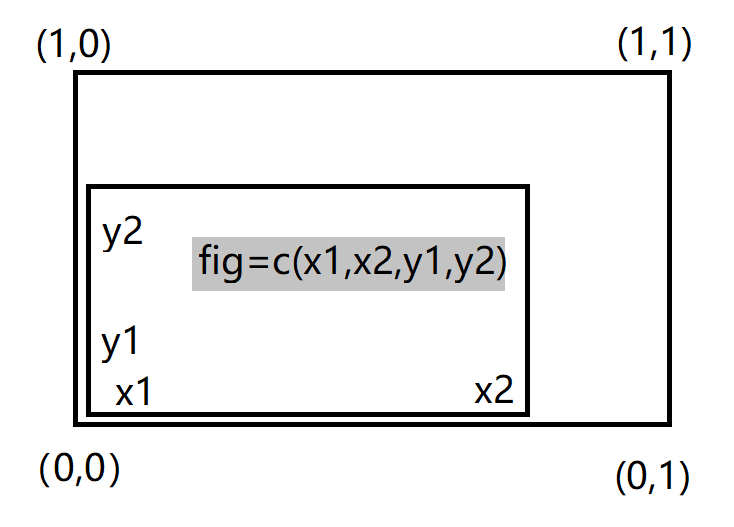opar <- par(no.readonly = TRUE)

par(fig=c(0,0.8,0,0.8)) # 设置散点图的布局参数

plot(iris$Sepal.Length,iris$Sepal.Width) #绘制散点图​

par(fig=c(0,0.8,0.65,1),new = TRUE) #设置上方箱型图的布局参数

boxplot(iris$Sepal.Length, horizontal = TRUE, axes=FALSE) # 绘制上方箱型图​ par(fig=c(0.65,1,0,0.8),new = TRUE) #设置右侧箱型图的布局参数 boxplot(iris$Sepal.Width, axes=FALSE) # 绘制右侧箱型图​

mtext("par(fig=c(x1, x2, y1, y2), new = TRUE)",side = 3, outer=TRUE, line=-3)

par(opar)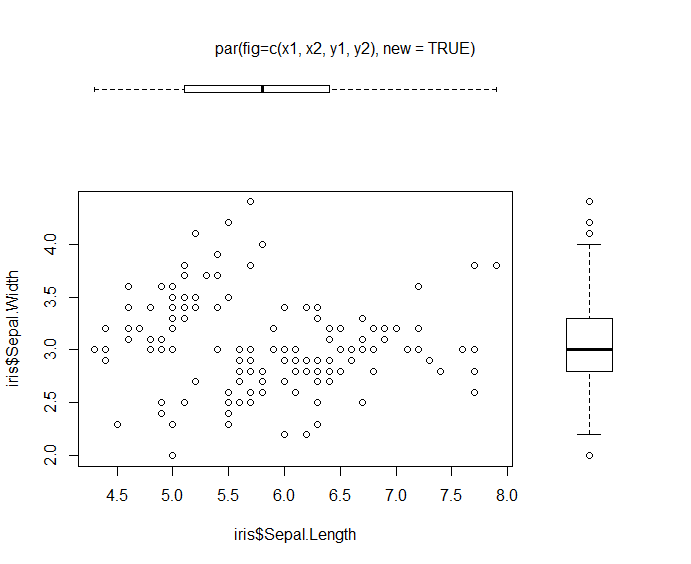03

—

图形组合布局

grid.layout & vplayout

library(ggplot2)

​#绘制基本ggplot图

base <- ggplot(mpg, aes(displ, hwy)) + geom_point()

p1 <- base + geom_smooth() + labs(title="图1") #如图1

​#用%+%调整映射关系中的数据

base <- ggplot(mpg, aes(displ, hwy)) + geom_point()

p2 <- base %+% subset(mpg, fl == "p") + labs(title="图2") #图2​#第二种调整数据的方法list

p3 <- base + list(subset(mpg, fl == "p"), geom_smooth(), labs(title="图3")) #图3​

###########一页多图########

library(grid)

grid.newpage()  ##新建页面

pushViewport(viewport(layout = grid.layout(2,2))) #将页面分成2*2矩阵

vplayout <- function(x,y){ viewport(layout.pos.row = x, layout.pos.col = y)}​

print(p1, vp = vplayout(1,1))   #（1,1)的位置画图1

print(p2, vp = vplayout(1,2))   #(1,2)的位置画图2

print(p3, vp = vplayout(2,1:2))  #（2,:)的位置画图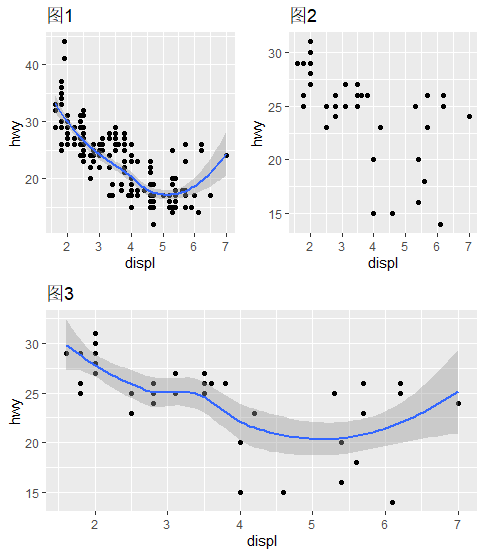04

—

图形组合布局

plot_grid {cowplot}

install.packages("cowplot") #安装cowplot包

library(cowplot) # 加载

?plot_grid  #帮助函数查看具体usage

示例

library(ggplot2)​

df <- data.frame(  x = 1:10, y1 = 1:10, y2 = (1:10)^2, y3 = (1:10)^3, y4 = (1:10)^4)

​p1 <- ggplot(df, aes(x, y1)) + geom_point()

p2 <- ggplot(df, aes(x, y2)) + geom_point()

p3 <- ggplot(df, aes(x, y3)) + geom_point()

p4 <- ggplot(df, aes(x, y4)) + geom_point()

gridplot_grid(p1, p2, p3, p4)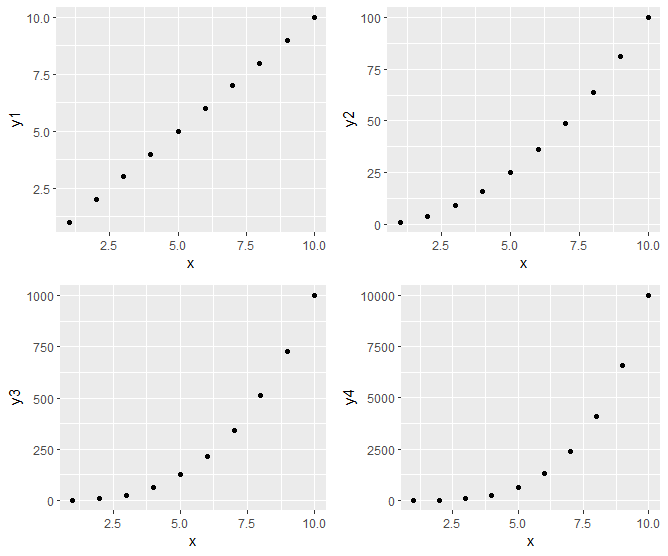05

—

图形组合布局

multiplot{Rmisc}

############################# multiplot{Rmisc} ##################################

library(Rmisc)

library(ggplot2)​

df <- data.frame(  x = 1:10, y1 = 1:10, y2 = (1:10)^2, y3 = (1:10)^3, y4 = (1:10)^4)​

p1 <- ggplot(df, aes(x, y1)) + geom_point()

p2 <- ggplot(df, aes(x, y2)) + geom_point()

p3 <- ggplot(df, aes(x, y3)) + geom_point()

p5 <- ggplot(mpg, aes(as.factor(year), hwy)) +  geom_boxplot() +  facet_wrap(~class, scales = "free_y")​

multiplot(p1, p2, p3, p5, cols=2)​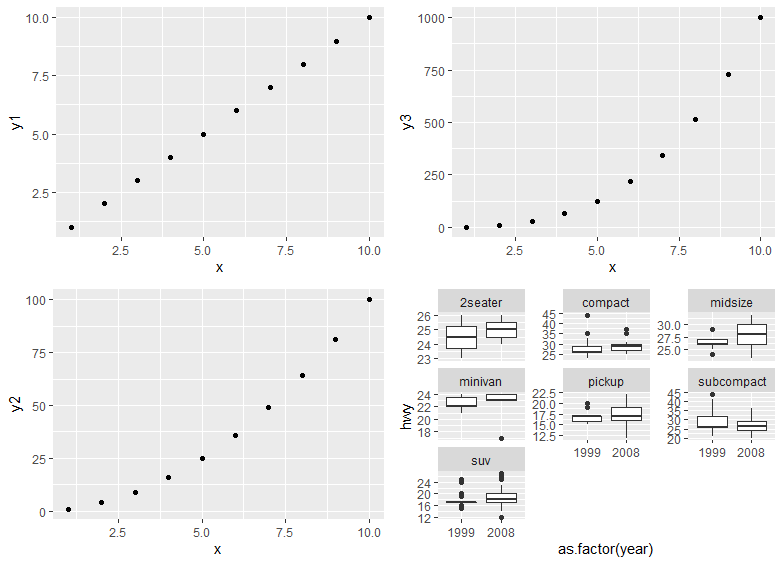“ 获取源代码请至“数据驱动实践”公众号后台回复：一页多图”


展开全文可视化
• R中使用函数par()或layout()可以容易地组合多幅图形为一幅总括图形。 你可以在par()函数中使用图形参数mfrow=c(nrows, ncols)来创建按行填充的、行数为 nrows、列数为ncols的图形矩阵。另外，可以使用nfcol=c(n...
备注：学习备忘
在R中使用函数par()或layout()可以容易地组合多幅图形为一幅总括图形。
你可以在par()函数中使用图形参数mfrow=c(nrows, ncols)来创建按行填充的、行数为
nrows、列数为ncols的图形矩阵。另外，可以使用nfcol=c(nrows, ncols)按列填充矩阵。
> attach(mtcars)
> opar<-par(no.readonly=T)
> par(mfrow=c(2,2))
> plot(wt,mpg,main="Main Title")
> plot(wt,disp,main="Main Tile1")
> hist(wt,main="main tile2")
> boxplot(wt,main="main title3")
> par(opar)
> detach(mtcars)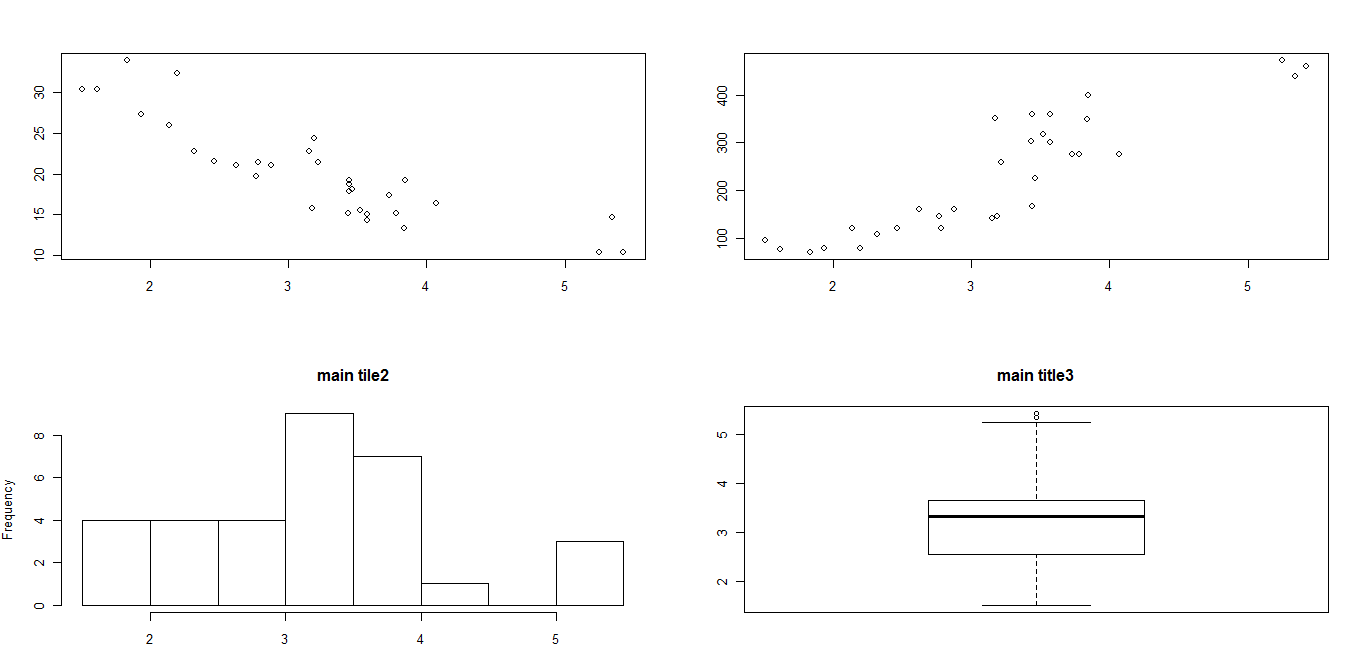函数layout()的调用形式为layout(mat)，其中的mat是一个矩阵，它指定了所要组合的
多个图形的所在位置。在以下代码中，一幅图被置于第1行，另两幅图则被置于第2行：
attach(mtcars)
layout(matrix(c(1,1,2,3),2,2,byrow=T))
hist(wt)
hist(mpg)
hist(disp)
detach(mtcars)精确地控制每幅图形的大小，可以有选择地在layout()函数中使用widths=和
heights=两个参数。其形式为：
widths = 各列宽度值组成的一个向量
heights = 各行高度值组成的一个向量
相对宽度可以直接通过数值指定，绝对宽度（以厘米为单位）可以通过函数lcm()来指定。
detach(mtcars)
> attach(mtcars)
> layout(matrix(c(1,1,2,3)2,2,byrow=T),widths=c(3,1),heights=c(1,2))
错误: 意外的数值量在"layout(matrix(c(1,1,2,3)2"里
> layout(matrix(c(1,1,2,3),2,2,byrow=T),widths=c(3,1),heights=c(1,2))
> hist(wt)
错误于plot.new() : 图版边太大
> par(mfrow=c(1,1))
> layout(matrix(c(1,1,2,3),2,2,byrow=T),widths=c(3,1),heights=c(1,2))
> hist(wt)
错误于plot.new() : 图版边太大
> layout(matrix(c(1,1,2,3),2,2,byrow=T),widths=c(3,1),heights=c(1,2))
> hist(wt)
错误于plot.new() : 图版边太大
> par(mar=c(5,4,4,2)+0.1)
> layout(matrix(c(1,1,2,3),2,2,byrow=T),widths=c(3,1),heights=c(1,2))
> hist(wt)
错误于plot.new() : 图版边太大
> hist(wt)
错误于plot.new() : 图版边太大
> par(mar=c(5,4,4,2)+0.1)
> hist(wt)
错误于plot.new() : 图版边太大
> par(mar=c(2,2,2,2)+0.1)
> hist(wt)
> hist(mpg)
> hist(disp)
> detach（）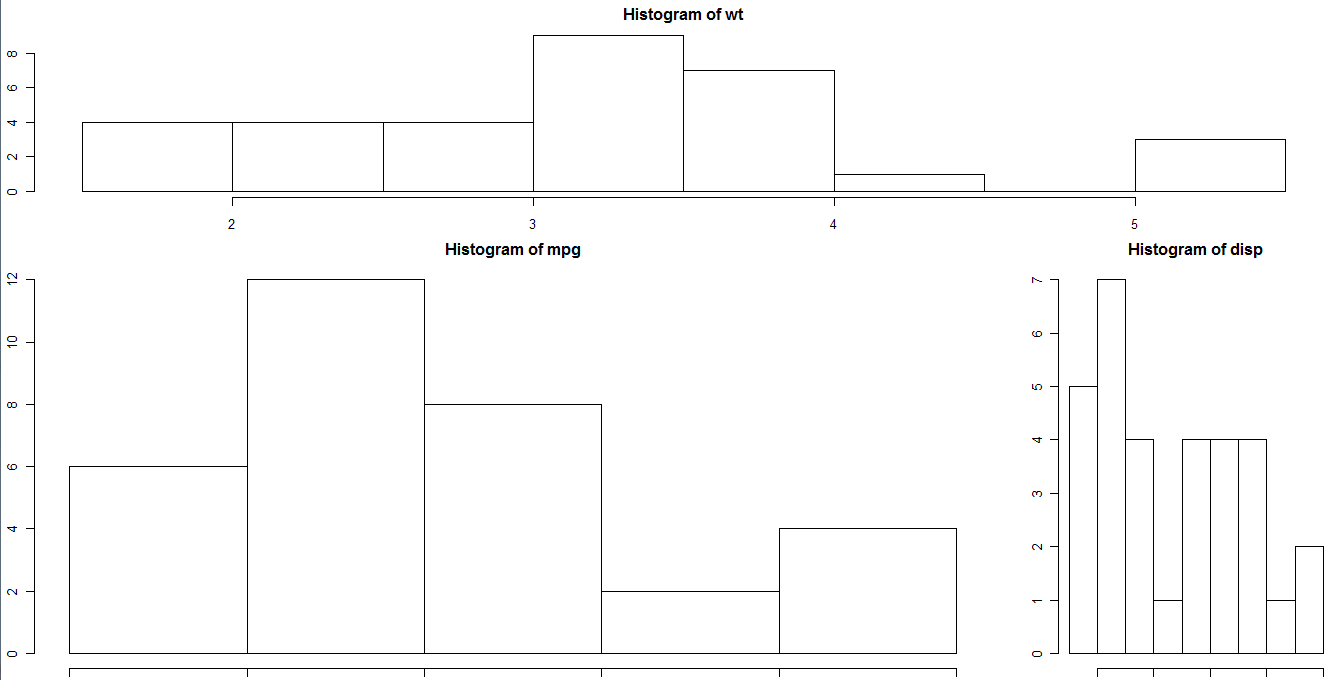展开全文• 相较于数字和文字的表示，图形的展示更能引起客户的兴趣，可视化程度高，通过看图我们得以发现数据中的模式或是检查出数据中的异常值，也有助于在数以千计的零散... 组合多个图形 1.1 图形的创建 注：可以通过代...
相较于数字和文字的表示，图形的展示更能引起客户的兴趣，可视化程度高，通过看图我们得以发现数据中的模式或是检查出数据中的异常值，也有助于在数以千计的零散信息中做出有意义的对比，提炼出使用其他方法时不那么容易发现的模式。

1、R图形需要掌握的基本要领：

图形的创建
图形的保存
自定义符号、线条、颜色和坐标轴
标注文本和标题
控制图形维度
组合多个图形
1.1 图形的创建

注：可以通过代码或图形用户界面来保存图形。要通过代码保存图形，将绘图语句夹在开启目标图形设备的语句和关闭目标图形设备的语句之间即可

创建一幅新图形时，通常会覆盖掉先前的图形。如何才能创建多个图形呢？创建一幅新图形之前打开一个新的图形窗口

dev.new()

….(描述图形)

dev,new()

….(描述图形)

如何查看？？

1）通过图形用户界面来查看多个图形

勾选“历史”（History）→“记录”（Recording）。然后使用菜单中的“上一个”（Previous）和“下一个”（Next）来逐个查看已经绘制的图形

2）使用函数dev.new()、dev.next()、dev.prev()、dev.set()和dev.off()同时打开多个图形窗口，并选择将哪个输出发送到哪个窗口中。关于这种方法的更多细节，请参考help(dev.cur)。

plot(x = x变量,
y = y变量,
type= "类型"
main="标题",
sub="子标题",
xlab="x轴名称",
ylab="y轴名称",
asp=0.1)  #y/x的比例，y轴数值长度与x轴数值长度的比值

关于type:
"p" for points, 散点图
"l" for lines, 折线图
"b" for both, 点线图
"c" for the lines part alone of "b", 只有线没有点，线段构成的图
"o" for both ‘overplotted’, 有线有点，且线穿过点
"h" for ‘histogram’ like (or ‘high-density’) vertical lines, 类似直方图，但是只有竖线
"s" for stair steps, 阶梯图
"S" for other steps,
"n" for no plotting. 只有坐标，没有图像

##绘图参数设置函数par()
参数：
pch:指定绘制点时使用的符号
cex:指定符号的大小，默认为1,1.5表示放大1.5倍0.5表示缩小为50%
lty:指定线型类型
lwd:指定线条宽度，默认1，lwd=2则线条宽度为默认值的2倍
no.readonly=TRUE/FALSE：假如=TRUE且没有其他变量， 则返回当前绘图设备下已设定好的绘图参数
ann= FALSE：不进行绘图解释标记（例如横轴标题等）
bg：设置绘图区背景色。缺省为bg = "transparent"（透明）
注意：某些高级绘图函数已经包含了默认的标题和标签。你可以通过在plot()语句或单独
的par()语句中添加ann=FALSE来移除它们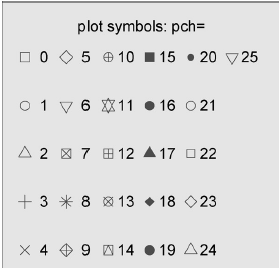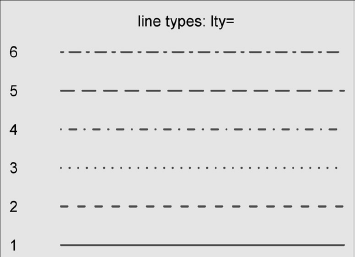1.2 图形的保存

pdf(“name.pdf”)    ##将图形保存到当前工作目录中名为name.pdf的PDF文件中（也可通过界          面保存） ##除了pdf()，还可以使用函数win.metafile()、png()、jpeg()、bmp()、tiff()、xfig()和postscript()将图形保存为其他格式

setwd("d://")
pdf("haha.pdf")##还是在用户保存来的方便，保存为png,emf
attach(mydata)
mydata
plot(a,b)
ablines(lm(b~a)） ##添加一条最优拟合曲线，LM算法，全称为Levenberg-Marquard算法
title("标题")
detach(mydata)
dev.off() ##结束图片编辑才行
getwd() ##可查看位置

1.3 图形的类型

lattice包提供了丰富的函数，可生成单变量图形（点图、核密度图、直方图、柱状图和箱线图）、双变量图形（散点图、带状图和平行箱线图）和多变量图形（三维图和散点图矩阵）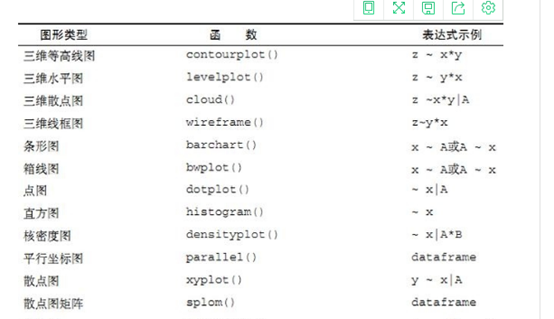1.4 图形颜色

在R中，可以通过颜色下标、颜色名称、十六进制的颜色值、RGB值或HSV值来指定颜色。举例来说，col=1、col="white"、col="#FFFFFF"、col=rgb(1,1,1)和col=hsv(0,0,1)都是表示白色的等价方式。函数colors()可以返回所有可用颜色的名称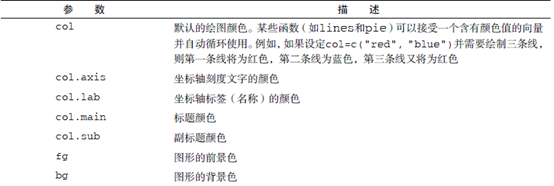1.5 坐标轴

par(font.lab=3,cex.lab=1.5,font.main=4,cex.main=2) ##之后创建的所有图形都将拥有斜体、1.5倍于默认文本大小的坐标轴标签（名称），以及粗斜体、2倍于默认文本大小的标题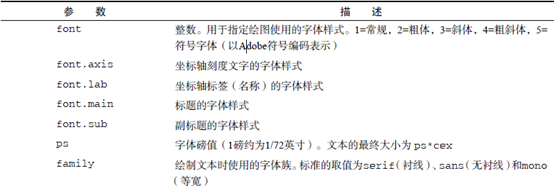1.6 尺寸大小

par(pin=c(4,3),mai=c(1,.5,1,.2)可生成一幅4英寸宽、3英寸高、上下边界为1英寸、左边界为0.5英寸、右边界为0.2英寸的图形。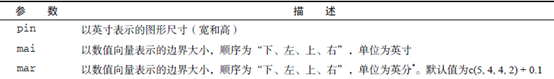1.7 添加文本、自定义坐标轴和图例

plot(xiaoguo,yao1,type="b",col="red",lty=2,pch=2,lwd=2,
main="clinical trains for yao1",sub="this is data",xlab="dasage",
ylab="response",xlim=c(0,60),ylim=c(0,70))

##还可以调用函数title()中指定图形的参数（如标题、坐标轴标签、文本大小、字体、旋转角度和颜色）
title(main="clinical trains for yao1",col.main="red",sub="this is data",col.sub="blue",
xlab="dasage",ylab="response",
col.lab="green",cex.lab=0.75)
##生成红色的标题和蓝色的副标题，以及较默认大小小25%的绿色x轴、y轴标签

1.8 图形的结合

在R中使用函数par()或layout()可以容易地组合多幅图形为一幅总括图，在par()函数中使用图形参数mfrow=c(nrows, ncols)来创建按行填充的、行数为nrows、列数为ncols的图形矩阵，用nfcol=c(nrows, ncols)按列填充矩阵。

##par组合
attach(mydata)
opar<-par(no.readonly=TRUE)
par(mfrow=c(2,2))
plot(a,b,main="title1")
plot(a,c,main="title2")
hist(a,main="title3") ##高级绘图函数hist()包含了一个默认的标题（使用main=""可以禁用它，抑或使用ann=FALSE来禁用所有标题和标签）
boxplot(a,main="title4")
par(opar)
detach(mydata)

##layout组合
attach(mydata)
layout(matrix(c(1,1,2,3),2,2,byrow=TRUE),widths=c(3,1),heights=c(1,2)

##1,1,2,3表示将屏幕切成3块，其排列类似于1123其中11部分部分是一张图，2是一张图，同样3也是一张图2,2表示将屏幕整体是分为两行两列的byrow=TRUE表示按行排图
##widths = 各列宽度值组成的一个向量
heights = 各行高度值组成的一个向量

hist(a)
hist(b)
hist(c)
detach(mydata)

1.9 一个例子

> xiaoguo<-c(20,30,40,50,60)
> yao1<-c(16,20,26,35,58)
> yao2<-c(13,16,23,31,39)
> opar<-par(no.readonly=TRUE) ##TRUE且没有其他变量， 则返回当前绘图设备下已设定好的绘图参数
> par(pin=c(2,3)) ##图形将为2英寸宽、3英寸高
> par(lwd=2,cex=1.5)
> par(cex.axis=.75,font.axis=3)
> plot(xiaoguo,yao1,type="b",pch=19,lty=2,col="red")
> plot(xiaoguo,yao2,type="b",pch=23,lty=6,col="green",bg="blue")
> par(opar)
##使用红色实心圆圈和虚线创建了第一幅图形，并使用蓝色菱形和绿色虚线创建了第二幅图形。最后，我们还原了初始的图形参数设置

##第二幅图会掩盖第一幅图
下面进行改善
> par(mfrow=c(1,2))
> plot(xiaoguo,yao1,type="b",pch=19,lty=2,col="red")
> plot(xiaoguo,yao2,type="b",pch=23,lty=6,col="green",bg="blue")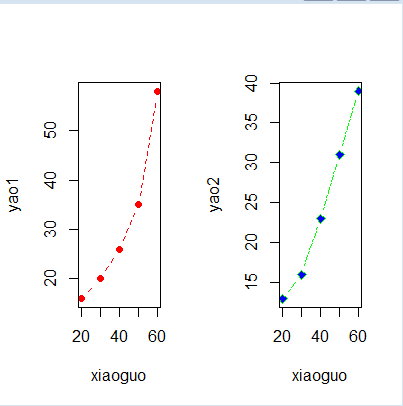2、plot、qplot、ggplot简单分析

三者作图建议参考https://blog.csdn.net/xiebin6163/article/details/70185970

##简单说明
plot(mydata$a,mydata$b)
qplot(a,b,data=mydata,geom='line'/c('line','point'))
##quick plot也可以qplot(mydata$a,mydata$b)
ggplot(mydata,aes(a,b)) +geom_要绘制的图形类型point or bar or...（）+labs()+…(可以加多个想要绘制的图形类型）
## geom_bar(stat = 'identity')  stat=’identity’y意为不对数据做统计运算，如果这里没有这步会报错
## geom_histogram(binwidth = 4) binwidth参数是调节横坐标的区间，你可以任意调节你认为合适的区间
## （柱状图）条形图与直方图看起来相似，但是却是不一样的，条形图的x轴是一个确定的数值，而直方图是一个区间
## geom_point(color = "blue") #设定散点的颜色为蓝色
## aes()函数是ggplot2中的映射函数, 所谓的映射即为数据集中的数据关联到相应的图形属性过程中一种对应关系
## labs()设置图片的标题, 子标题, 引用，x轴和y轴的标题
eg:labs(title = "sss", subtitle = "ss", caption = "s",xlab="a",ylab="b")



展开全文plot
• 1颜色设置 2 plot 的参数配置 3 图形尺寸和边界尺寸 4 坐标轴 5 图例 6 文本属性 7 多图多线 8 图形组合 9 交互图形函数 10散点图 11相关图 12箱线图 13饼图 14条形图 15直方图 1颜色设置-R 预设调色板 这一系列函数...
• 本文将接着讲解ggplot2的多图排列的下半部分，通过其他方式排列组合多张ggplot2的图。除此以外还可以给图加上图层，让你的数据报告更加多姿多彩。 整合多图 我们可以把不同类型的图放在一起，比方说散点图和密度图...可视化 数据可视化
• 使用R语言中的聚类是，当需要聚类的数据比较时，常常出现下面的图片。这时候我们怎么办法呢。 这是变量较少的时候的： 可是当变量比较就是这个样子了： 解决方式 数据处理 当遇到上述情况，可是又想可视化...聚类
• setwd("C:/Users/yuki_cool/RWorkspace") library(ggplot2) library(gridExtra) ##张图片组合为一张图片上 library(RColorBrewer) mypalette(7,"Reds") paste("aaa","dddd",sep = "") LSQ_data ("C:/Us
• R中使用函数par()，layout()可以很容易组合多副图为一副总括图形。 一、par() 用法 在par 函数中使用图形参数mfrow=c(nrows,ncols)来按照行填充的行数为nrows，列数为ncols的图形矩阵。也可以使用mfcol=c(nrows,...par layout fig
• 甚至很图形可以直接拿excel做（为了匹配PPT），但是R中的plot类图形不是很美观，并且最大的问题是很数据、图形和匹配无法分离，这样的话，如果针对一个数据做张图，或个数据做一张会有些费劲。在学习了一...
• 上一节探索了散点，但是散点好像可以编辑的点不，排列组合也不太；下面进行线图的探索1、 最简单的线图 还是借用mtcars的数据集：p(data=mtcars) p+geom_line(aes(x=wt,y=mpg))结果如： 也就是横轴为wt...
• 本节内容包括 ...组合多个图形 1.使用图形 pdf("mygraph.pdf")#保存pdf 也可以png/jpeg/bmp/tiff/xfig...attach(mtcars) plot(wt, mpg) abline(lm(mpg-wt)) #求出回归参数，并作出线 title("Re...
• 3.1.4 绘图尺寸、绘图区域、地图比例以及多图绘制 3.1.5 绘图属性和地图图例 3.2 使用spplot的trellis/lattice绘制 3.2.1 一个直观的trellis示例 3.2.2 绘制点、线、面和网格 3.2.3 对图添加参考物和布局元素 ...
• 组合多个图形 3.1 使用图形 创建个图形并随时查看每一个 1.创建一幅新图形之前，打开一个新的图形窗口 dev.new() statements to create new graph 2.通过图形界面勾选 3.dev.new/next/prev/set/off()
• R语言：我写的一个数据可视化的函数，散点和线性回归趋势线及公式在做项目的时候，发现需要次绘制散点和图例，以及计算线性回归的拟合公式和R2，为了方便处理，特编写了这个函数，希望对大家有所帮助。...
• 也欢迎大家提出报给的改进建议或者更的需求组合。具体的可视化函数如下。 其中，data.xts是tidy的时间数据集，调用时只需要给出time和lie这2个变量，lie的格式是data.xts的第几个字段。sandiantu函数直接绘制散点...
• R语言经典实例（中+英） 第1章 R入门和获得帮助 7 　1.1 下载和安装R软件 8 　1.2 开始运行R软件 10 　1.3 输入R命令 13 　1.4 退出R 15 　1.5 中断R正在运行的程序 16 　1.6 查看帮助文档 17 　1.7 获取函数的帮助...
• 1.展示名义变量和缺失值变量的关系 ...绘制不同季节的mxPH的直方，由于直方相似，所以收集样本时改年的季节对变量mxPH的值没有显著影响，可以用个名义变量进行组合 histogram(~mxPH|size*sp
• 初用R，发现在用基础包绘图时不同的画图函数中很参数都通用的，每次遇见都要去R中查help文件，所以在此借鉴论坛中优秀的par解说，整理成手册，加深自己记忆的同时，方便以后查找参数~ par参数涉及颜色、字体、...
• 以列表方式表示两个(或个)变量或属性共同出现的频率。 或者是将两个属性变量的不同取值置于行和列的位置，在表格中填入变量组合取值的频数的表格。 #描述性统计Fenix summary(stock) #最小值，最大值，中位数...
• 左图单个分类器就是上的对角线，当进行组合时，出现了上的折线，每个折边都是一个分类器，当有个分类器进行组合，就会出现右图，最终达到一个曲线组合算法的优势：1、能明显提升判别准确率；2、对...
• 昨天晚上看一本书,讲的是android中的设计模式,其中用到了很的uml, 虽然之前自己也会点, 但是, 也没有系统的学习过;...3. 说明类的名称必须是名词，不需要前缀或后缀，可以个名称组合，单词首字母android uml 设计模式
• ## PCA图

2019-10-06 21:19:50
R语言PCA 1、关键点 综述：主成分分析 因子分析 典型相关分析，三种方法的共同点主要是用来对数据降维处理的从数据中提取某些公共部分，然后对这些公共部分进行分析和处理。 #主成分分析 是将指标化为少数几...
• lattice继承了R语言的一个糟糕特征，就是参数设置铺天盖地，足以让人窒息，光是一份xyplot()函数的帮助文档，恐怕就够我们消磨一天时间了，更重要的是，lattice仍然面向特定的统计图形，像基础图形系统一样，有直方...
• 假设每个单词都包含一个或个词素，这些词素可以定义为具有特定含义或语法功能的最小语言单元。 一个词可以碰到形态单一的单词，即词根，也可以碰到形态复杂的单词，例如复合词或词缀形式。 Batı-lı-laş-tır-...
• 课程目录： 第1章: 数据分析师先导篇 任务1： 数据分析的概念 ...任务299： 为什么要学习R语言R语言的引入及R语言概述 任务300： R 安装、基本使用（HelloWorld程序及函数和对象初步认识） 任务...数据分析师
• 假设每个单词都包含一个或个词素，这些词素可以定义为具有特定含义或语法功能的最小语言单元。 一个词可以碰到形态单一的单词，即词根，也可以碰到形态复杂的单词，例如复合词或词缀形式。 Batı-lı-laş-tır-......